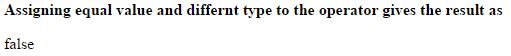# Equal value and Equal type (===) operator in JavaScript

### Equal value and Equal type (===)

Equal value and equal type operator is an comparison operator which is used to check the whether two operands are having same value and same type.

For example, in the Equal operator we can write same value in different types gives the same result, like we declared `var a = 5` and we are assigning `a == 5` or `a == "5"` to the opertor gives the same result, but in Equal value and Equal type operator it is not possible.

The symbolic representation of equal value and equal type is `===`.

### Assigning same type values

```<b>Assigning equal value and equal type to the operator gives the result as</b>
<p id="myId"></p>

<script>
var a = 30;
document.getElementById("myId").innerHTML = (a === 30);
</script>
```

In the above code snippet we have given same type of values to the variable `a` and to the operator, so the result give 'true'.

OUTPUT### Assigning same value in different types

```<b>Assigning equal value and differnt type to the operator gives the result as</b>
<p id="myId"></p>

<script>
var a = 30;
document.getElementById("myId").innerHTML = (a === "30");
</script>
```

Equal value and different type

In the above code snippet we have declared equal value to the variable `a` and to the operator, but we declared in different types, that means the value is given directly to the variable `a` and the value given to the operator is kept in inverted coma's, so the result gives 'false'.

OUTPUTViews: 9009 | Post Order: 169